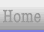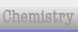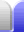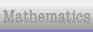# Series vs Parallel, Measuring Circuits

### Series & Parallel

A series circuit has components "one after the other" so that the same current goes through them all.This circuit has two resistors in series. The same current flows through both resistors. Voltmeters (each placed in parallel with a resistor) show that both resistors have the same voltage drop across them, and therefore have the same value.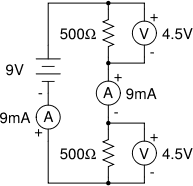A parallel circuit has the components "side by side" so that the total current has to split to go through them.This circuit has two resistors in parallel. The battery provides a total of 36mA. Because the resistors have the same value the current splits equally between them.### Measuring circuits

Current is measured with an ammeter. An ammeter is connected in series, to measure the current flowing through a particular point in the circuit. An ideal ammeter will have no resistance so no voltage will be dropped across the ammeter, which would affect the current flowing through the circuit. In practice ammeters do have a small amount of resistance, and ammeter leads also have their own resistance.

A galvanometer is a type of ammeter.

Voltage is measured with a voltmeter. A voltmeter is connected in parallel with the component it is measuring the voltage drop across. An ideal voltmeter will have infinite resistance so no current flows through the voltmeter instead of where it should go – through the component it is connected across.

Resistance is measured with an ohmmeter. The ohmmeter uses a small known current to measure the voltage drop across a component or circuit.

A multimeter can be used to measure all the above by selecting the desired range with a rotary switch.

Using Ohm's Law (V = IR) we only need to know any two out of voltage, current and resistance to be able to work out the third.Home Astronomy Chemistry Electronics Mathematics Physics Field Trips Turn on javascript for email link